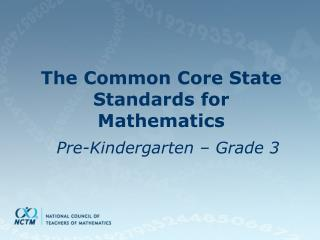DownloadDownload PresentationThe Common Core State Standards for Mathematics

# The Common Core State Standards for Mathematics

Télécharger la présentation## The Common Core State Standards for Mathematics

- - - - - - - - - - - - - - - - - - - - - - - - - - - E N D - - - - - - - - - - - - - - - - - - - - - - - - - - -
##### Presentation Transcript

1. The Common Core State Standards for Mathematics Pre-Kindergarten – Grade 3

2. Common Core Development • Initially 48 states and three territories signed on • As of December 1, 2011, 45 states have officially adopted • Final Standards released June 2, 2010, can be downloaded at www.corestandards.org • Adoption required for Race to the Top funds

3. Preschool • There are no PreK CCSSM standards

4. Kindergarten • The Standards do not dictate curriculum or teaching methods. Teachers have the autonomy to use each Standard as they see fit. • Expectations that begin with the word “understand” are good opportunities to connect the practice to the content. • Two critical areas of focus: • Representing, relating, and operating on whole numbers with sets of numbers • Describing shapes and space

5. Kindergarten • Representing, Relating, and Operating on Whole Numbers • Counting and cardinality • Know numbers’ names and the count sequence • Count to tell the number of objects • Compare numbers • Operations and Algebraic Thinking • Understand addition and subtraction • Putting together and adding to • Taking apart and taking from • Fact families up to 10

6. Kindergarten • Place Value 11-19 • 15 = ten ones and five ones • Measurable attributes • Length and weight • Classify objects

7. Kindergarten • Geometry • Identify and describe shapes • 2D and 3D shapes

8. Kindergarten: What’s Different • No time • No calendar • No graphing • No money • No non-standard measurement • No fractions • No patterning • No estimation

9. First Grade • Four critical areas: • Developing understanding of addition and subtraction, and strategies for addition and subtraction within 20 • Whole number relationships and place value • Linear measurement and measuring lengths • Composing and decomposing geometric shapes

10. First Grade • Geometry • Reason with shapes and their attributes • 2D and 3D shapes

11. First Grade • Operations and Algebraic Thinking • Addition and subtraction within 20 • Apply operations: commutative and associative • Number and Operations in Base Ten • Measure lengths indirectly and by iterating length units • Time (hour and half hour) • Represent and interpret data • Fractions halves, fourths, quarters

12. First Grade: What’s Different • No money • No standard units of measure • No patterning • No estimation

13. SecondGrade • Four critical areas: • Extending understanding of base-ten notations • Building fluency with addition and subtraction • Using standard units of measure • Describing and analyzing shapes

14. Second Grade • Operations and Algebraic Thinking • Represent and solve problems involving addition and subtraction within 100 to solve one- and two-step word problems • Add and subtract within 20 • Work with equal groups of objects to gain foundation for multiplication

15. Second Grade • Number and Operations in Base Ten • Understand place value – 3 digits, count within 1,000, compare two three-digit numbers • Use place value understanding and properties of operations to add and subtract • Measurement and Data • Measure and estimate lengths in standard units • Relate addition and subtraction to length • Work with time and money • Draw picture graphs and bar graphs

16. Second Grade • Geometry • Reason with shapes and their attributes: angles, faces, quadrilaterals, pentagons, hexagons, and cubes • Fractions (whole, halves, thirds)

17. Second Grade: What’s Missing • No patterns • No 2D or 3D shapes • No estimation

18. Third Grade • Four critical areas • Multiplication and division and strategies for multiplication and division within 100 • Develop understanding of fractions, especially unit fractions • The structure of rectangular arrays and of area • Describe and analyze 2D shapes

19. Third Grade • Operations and Algebraic Thinking • Represent and solve problems involving multiplication and division • Commutative property of multiplication • Multiply and divide within 100 • Solve problems involving the four operations and identify and explain patterns in arithmetic

20. Third Grade • Number and Operations in Base Ten • Number and Operations – Fractions • Develop understanding of fractions as numbers • Measurement and Data • Time to the minute • Measure and estimate liquid volumes and masses of objects using grams, kilograms, and liters • Represent and interpret data

21. ThirdGrade • Geometric measurement: • Understand concepts of area and relate area to multiplication and division • Recognize perimeter as an attribute of plane figures and distinguish between linear and area measures • Geometry • Reason with shapes and their attributes

22. Third Grade: What’s Different • No line symmetry • Omits solids and looking at objects from different viewpoints; transformations and congruence